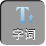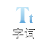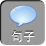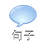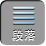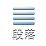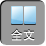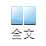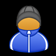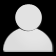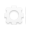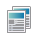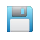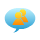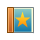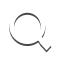-AA+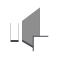1如图 1，在 RtABC中，∠ B= 90°， AB= 3BC= 4，将△ ABC折叠，使点 B恰好落在斜边 AC上，与点 B′重合， AE为折痕，则 EB′的长为.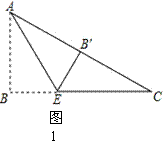BE= EB′= x，则 EC= 4 - x.

RtABC中， AB= 3BC= 4，由勾股定理，得 AC=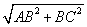=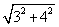= 5.

BC= 5-3= 2.

RtBEC中，由勾股定理，得 x 2+ 2 2=( 4 - x) 2解得 x= 1.5.

2( 2015年江苏省泰州市中考试题)如图 2，在长方形 ABCD中， AB= 8BC= 6PAD上一点，将△ ABP沿 BP翻折至△ EBPPECD相交于点 O，且 OE= OD，则 AP的长为_______.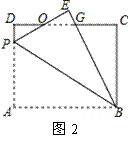∴∠ D=∠ A=∠ C= 90°， AD= BC= 6CD= AB= 8.

∴△ ODP≌△ OEG. OP= OGPD= GE.

OP+ OE= OD+ OG，即 DG= EP.

AP= EP= x，则 PD= GE= 6 - xDG= x

CG= 8 - xBG= 8 -( 6 - x)= 2+ x.

RtBCG中，由勾股定理，得 BC 2+ CG 2= BG 2

6 2+( 8 - x) 2=( x+ 2) 2 解得 x= 4.8，即 AP= 4.8.

【温馨提示】图形的翻折的实质是图形的全等，解题时要注意寻找出折叠前后的不变量(即相等的线段、相等的角)；应该在图形中寻求最佳的直角三角形，运用勾股定理创设方程模型来解题；有时还得需要作出合理的辅助线来构造直角三角形∴∠ DAC60°－ 30°＝ 30°， DEDC.

RtDCA中， DC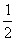DA.

DCx㎝，则 DA2 x㎝，

RtDAC中，根据勾股定理，得 DC 2CA 2DA 2，即 x 29＝( 2 x) 2.

x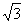DEDC